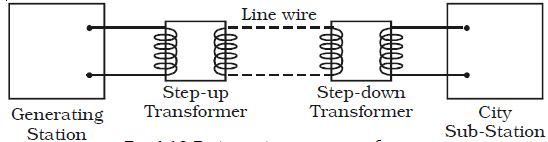Physics

# Describe on Long Distance Power Transmission

Long distance power transmission

The electric power generated in a power station situated in a remote place is transmitted to different regions for domestic and industrial use. For long distance transmission, power lines are made of conducting material like aluminium. There is always some power loss associated with these lines.Fig: Distance transmission of power

If I is the current through the wire and R the resistance, a considerable amount of electric power I2R is dissipated as heat.

Hence, the power at the receiving end will be much lesser than the actual power generated. However, by transmitting the electrical energy at a higher voltage, the power loss can be controlled as is evident from the following two cases.

Case (i) A power of 11,000 W is transmitted at 220 V.

Power P = VI

∴ I = P/V = 11,000/220 = 50A

If R is the resistance of line wires,

Power loss = I2R = 502R = 2500(R) watts

Case (ii) 11,000 W power is transmitted at 22,000 V

∴ I = P/V = 11,000/22,000 = 0.5 A

Power loss = I2R = (0.5)2 R = 0.25(R) watts

Hence it is evident that if power is trasmitted at a higher voltage the loss of energy in the form of heat can be considerably reduced.